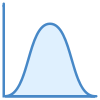Category: Helper Functions# syRandomNormal

Returns random real number according to normal distribution. A new random real number is returned every time the worksheet is calculated.

## Syntax

`syRandomNormal(Mean, StDev)`

The syRandomDiscrete function syntax has the following arguments:

• Mean: required. Mean of the distribution.
• StDev: required. Standard deviation of the distribution.

Return value is real number generated according to the normal distribution and given arguments.

## Examples

• `syRandomNormal(3.23, 1.57)` - The function draws real numbers according to the function arguments.